International
Tables for
Crystallography
Volume B
Reciprocal space
Edited by U. Shmueli

International Tables for Crystallography (2006). Vol. B, ch. 5.1, pp. 543-544   | 1 | 2 |

## Section 5.1.6.4. Reflecting power

A. Authiera*

aLaboratoire de Minéralogie-Cristallographie, Université P. et M. Curie, 4 Place Jussieu, F-75252 Paris CEDEX 05, France
Correspondence e-mail: authier@lmcp.jussieu.fr

#### 5.1.6.4. Reflecting power

| top | pdf |

For an absorbing crystal, the intensities of the reflected and refracted waves are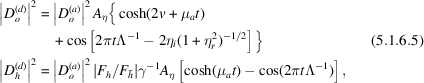where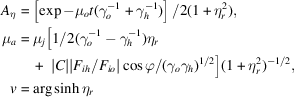and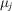is given by equation (5.1.6.1).

Depending on the absorption coefficient, the cosine terms are more or less important relative to the hyperbolic cosine term and the oscillations due to Pendellösung have more or less contrast.

For a non-absorbing crystal, these expressions reduce to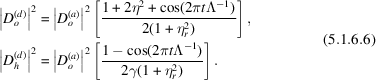What is actually measured in a counter receiving the reflected or the refracted beam is the reflecting power, namely the ratio of the energy of the reflected or refracted beam on the one hand and the energy of the incident beam on the other. The energy of a beam is obtained by multiplying its intensity by its cross section. If l is the width of the trace of the beam on the crystal surface, the cross sections of the incident (or refracted) and reflected beams are proportional to (Fig. 5.1.6.5)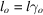and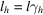, respectively.Figure 5.1.6.5 | top | pdf |Cross sections of the incident,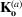, refracted,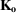, and reflected,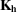, waves.

The reflecting powers are therefore: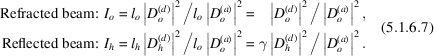Using (5.1.6.6), it is easy to check that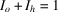in the non-absorbing case; that is, that conservation of energy is satisfied. Equations (5.1.6.6)show that there is a periodic exchange of energy between the refracted and the reflected waves as the beam penetrates the crystal; this is why Ewald introduced the expression Pendellösung.

The oscillations in the rocking curve were first observed by Lefeld-Sosnowska & Malgrange (1968, 1969). Their periodicity can be used for accurate measurements of the form factor [see, for instance, Bonse & Teworte (1980)]. Fig. 5.1.6.6shows the shape of the rocking curve for various values of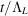.Figure 5.1.6.6 | top | pdf |Theoretical rocking curves in the transmission case for non-absorbing crystals and for various values of: (a)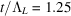; (b)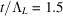; (c)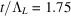; (d)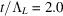.

The width at half-height of the rocking curve, averaged over the Pendellösung oscillations, corresponds in the non-absorbing case to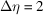, that is, to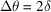, where δ is given by (5.1.3.6).

### References

Bonse, U. & Teworte, R. (1980). Measurement of X-ray scattering factors of Si from the fine structure of Laue case rocking curves. J. Appl. Cryst. 13, 410–416.Google Scholar
Lefeld-Sosnowska, M. & Malgrange, C. (1968). Observation of oscillations in rocking curves of the Laue reflected and refracted beams from thin Si single crystals. Phys. Status Solidi, 30, K23–K25.Google Scholar
Lefeld-Sosnowska, M. & Malgrange, C. (1969). Experimental evidence of plane-wave rocking curve oscillations. Phys. Status Solidi, 34, 635–647.Google Scholar